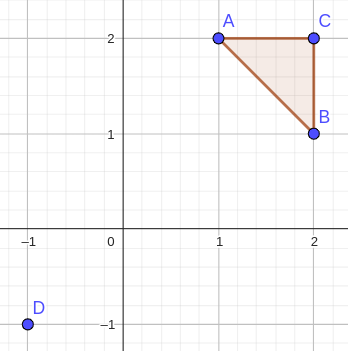# Profitable HomeNirjhorCoU-BRACNet Inter Univers...3/4/23
Limits 4s, 64 MB

Milk and Mocha want to move to a new house in Coffeeland. They don’t like ugly corners sticking into their house, so they want the shape of the house to be a convex polygon with a positive area. Mocha has already shortlisted $N$ distinct points where the pillars of the house can be placed at. They just need to choose a few of them and build walls around them.

The transactions in Coffeeland happen with coffee beans. Mocha has already contacted a construction company to build the walls for them. The company doesn’t charge for the pillars. To build a wall between two pillars, the company charges the number of coffee beans equal to the squared Euclidean distance between the pillars. The cost to build a house would therefore be the sum of the costs to build each such wall.

Milk and Mocha like open spaces. However, financially they’re very aware. They’d like to get the best out of their investment. That’s why, they’d choose a house that maximizes the area per every coffee bean they’d be investing. Can you find the maximum possible value for them? You need to do it for $T$ construction sites that they have in mind.

## Input

The first line contains $T$, the number of independent construction sites Milk and Mocha have in mind. Then the descriptions of these $T$ cases follow.

The first line of each case contains the integer $N$, the number of points where pillars can be placed. This number satisfies $3\leq N\leq 200$.

The next $N$ lines contain two space-separated integers $x$ and $y$ each, denoting the coordinates of the chosen points. These numbers satisfy $-1000\leq x,y\leq 1000$. No three of these $N$ points are collinear.

The total number of points over all these cases does not exceed $200$.

## Output

For each case, print a single real number — the maximum possible value of area per coffee bean they can get if they choose their house wisely.

Your answer would be considered correct if the absolute difference to the actual answer is within $10^{-6}$.

## Sample

InputOutput
2
4
1 2
2 1
2 2
-1 -1
4
0 0
5 0
0 5
5 5

0.125
0.25


The points in the first sample case represent the points $A,B,C,D$ respectively in the following image.One can check that it’s the most profitable to build a house around the triangle $ABC$, giving us the value of $\dfrac{\triangle ABC}{|AB|^2+|BC|^2+|CA|^2}=\dfrac{0.5}{(1^2+1^2)+(0^2+1^2)+(1^2+0^2)}=0.125$ for area per coffee bean. Here they get an area of $0.5$ for $4$ coffee beans.

In particular, choosing to build a house around all the points (i.e. choosing $ADBC$) brings them a bigger area, but also costs a lot more, leading to a smaller value of area per coffee bean.

The points in the second sample are the vertices of a square, and building the house around all the points is one optimal solution.

### Submit